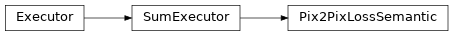# Pix2PixLossSemantic¶

Inheritance Diagramclass ashpy.losses.gan.Pix2PixLossSemantic(cross_entropy_weight=100.0, adversarial_loss_weight=1.0, feature_matching_weight=10.0, adversarial_loss_type=<AdversarialLossType.GAN: 1>, use_feature_matching_loss=False)[source]

Semantic Pix2Pix Loss.

Weighted sum of ashpy.losses.gan.CategoricalCrossEntropy, ashpy.losses.gan.AdversarialLossG and ashpy.losses.gan.FeatureMatchingLoss.

Methods

 __init__([cross_entropy_weight, …]) Initialize the Executor.

Attributes

 executors Return the List of Executors. fn Return the Keras loss function to execute. global_batch_size Global batch size comprises the batch size for each cpu. weight Return the loss weight.
__init__(cross_entropy_weight=100.0, adversarial_loss_weight=1.0, feature_matching_weight=10.0, adversarial_loss_type=<AdversarialLossType.GAN: 1>, use_feature_matching_loss=False)[source]

Initialize the Executor.

Weighted sum of ashpy.losses.gan.CategoricalCrossEntropy, ashpy.losses.gan.AdversarialLossG and ashpy.losses.gan.FeatureMatchingLoss

Parameters: cross_entropy_weight (ashpy.ashtypes.TWeight) – Weight of the categorical cross entropy loss. adversarial_loss_weight (ashpy.ashtypes.TWeight) – Weight of the adversarial loss. feature_matching_weight (ashpy.ashtypes.TWeight) – Weight of the feature matching loss. adversarial_loss_type (ashpy.losses.gan.AdversarialLossType) – type of adversarial loss, see ashpy.losses.gan.AdversarialLossType use_feature_matching_loss (bool) – whether to use feature matching loss or not